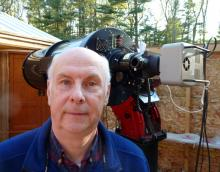# BSM_NH2b Transformation Coefficients

1 post / 0 new
MZKBSM_NH2b Transformation Coefficients

Attached are transformation coefficients for the BSM_NH2b system which includes an E180 scope and ASI183 CMOS camera. The SR filter has been transformed to a Cousins R filter.

[Setup]
description= TG - Version TG_V7.4, Telescope= BSM_NH2b, Time created (UT) = 2020_05_25_15:31:59
[Coefficients]
Tbv= 1.021
Tb_bv= -0.053
Tv_bv= -0.067
Tvr= 1.179
Tv_vr= -0.119
Tr_vr= -0.283
Tri= 0.836
Tr_ri= -0.334
Ti_ri= -0.116
Tvi= 1.004
Tv_vi= -0.063
Ti_vi= -0.055
Tr_vi= -0.150
[Error]
Tbv= 0.008
Tb_bv= 0.008
Tv_bv= 0.008
Tvr= 0.015
Tv_vr= 0.014
Tr_vr= 0.022
Tri= 0.012
Tr_ri= 0.023
Ti_ri= 0.037
Tvi= 0.011
Tv_vi= 0.007
Ti_vi= 0.016
Tr_vi= 0.011
[R Squared Values]
Tbv= 0.994
Tb_bv= 0.340
Tv_bv= 0.386
Tvr= 0.984
Tv_vr= 0.406
Tr_vr= 0.739
Tri= 0.991NH2b Transform NGC7790 200525.txt